#### You may also like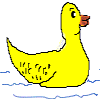### Dining Ducks

Use the information about the ducks on a particular farm to find out which of the statements about them must be true.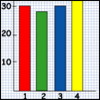### How Big Are Classes 5, 6 and 7?

Use the two sets of data to find out how many children there are in Classes 5, 6 and 7.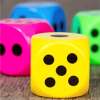### You Never Get a Six

Charlie thinks that a six comes up less often than the other numbers on the dice. Have a look at the results of the test his class did to see if he was right.

# Graphing Number Patterns

## Graphing Number Patterns

The class were making number patterns and then making graphs of them.

Several children had plotted graphs of the "times tables". They made good-looking straight lines.

Tom had started on the six times table but had then decided to do something more interesting. He had made the triangular numbers with counters last year. That was a better idea, he thought.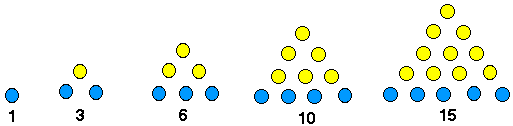So he started to put them on the same graph paper as the unfinished six times table.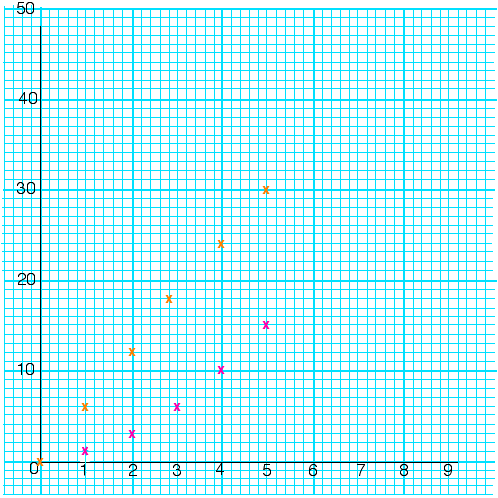"It's not a very good straight line," he remarked to Andy who was sitting next to him.
"I think it's going to cross the six times line," answered Tom, "But you'll have to make a lot more of both of them. I'm going to try square numbers, I bet the tables one will cross that!"

Does the graph of the triangular numbers cross that of the six times table?
And if it does, where?

Does the graph of square numbers cross those of the times tables?
And if it does, where?

### Why do this problem?

This problem can be done when the group are occupied with making straight-line graphs of multiplication tables and similar material. It could just nudge certain learners into something new. If they do not know the triangular numbers they could be shown how to work them out first. Drawing the graphs on paper is most satisfactory, although a graphing calculator or a computer can be used.

### Key questions

Is this a straight-line graph?
Which times tables have you done?
Do you know the figures you need for the triangular numbers?
What about the square numbers? How far do you think you will be able to get on that paper?

### Possible extension

Learners could try sequences such as doubling or adding successively one, two, three. Alternatively this Matchsticks problem could be used.

### Possible support

Some children might benefit from drawing the graphs of multiplication tables on paper. A graphing calculator or on a computer can also be used.